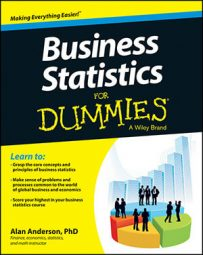You can use the Central Limit Theorem to convert a sampling distribution to a standard normal random variable. Based on the Central Limit Theorem, if you draw samples from a population that is greater than or equal to 30, then the sample mean is a normally distributed random variable. To determine probabilities for the sample mean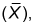the standard normal tables requires you to convert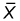to a standard normal random variable.

The standard normal distribution is the special case where the mean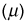equals 0, and the standard deviation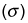equals 1.

For any normally distributed random variable X with a mean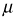and a standard deviation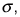you find the corresponding standard normal random variable (Z) with the following equation: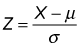For the sampling distribution of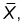the corresponding equation is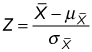As an example, say that there are 10,000 stocks trading each day on a regional stock exchange. It's known from historical experience that the returns to these stocks have a mean value of 10 percent per year, and a standard deviation of 20 percent per year.

An investor chooses to buy a random selection of 100 of these stocks for his portfolio. What's the probability that the mean rate of return among these 100 stocks is greater than 8 percent?

The investor's portfolio can be thought of as a sample of stocks chosen from the population of stocks trading on the regional exchange. The first step to finding this probability is to compute the moments of the sampling distribution.

• Compute the mean: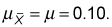The mean of the sampling distribution equals the population mean.
• Determine the standard error: This calculation is a little trickier because the standard error depends on the size of the sample relative to the size of the population. In this case, the sample size (n) is 100, while the population size (N) is 10,000. So you first have to compute the sample size relative to the population size, like so: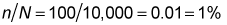Because 1 percent is less than 5 percent, you don't use the finite population correction factor to compute the standard error. Note that in this case, the value of the finite population correction factor is: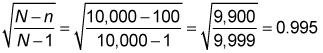Because this value is so close to 1, using the finite population correction factor in this case would have little or no impact on the resulting probabilities.

And because the finite population correction factor isn't needed in this case, the standard error is computed as follows: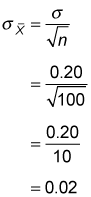To determine the probability that the sample mean is greater than 8 percent, you must now convert the sample mean into a standard normal random variable using the following equation:To compute the probability that the sample mean is greater than 8 percent, you apply the previous formula as follows: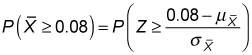Because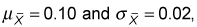these values are substituted into the previous expression as follows: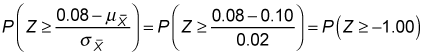You can calculate this probability by using the properties of the standard normal distribution along with a standard normal table such as this one.

Standard Normal Table — Negative Values
Z 0.00 0.01 0.02 0.03
–1.3 0.0968 0.0951 0.0934 0.0918
–1.2 0.1151 0.1131 0.1112 0.1093
–1.1 0.1357 0.1335 0.1314 0.1292
–1.0 0.1587 0.1562 0.1539 0.1515
The table shows the probability that a standard normal random variable (designated Z) is less than or equal to a specific value. For example, you can write the probability that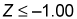(one standard deviation below the mean) as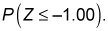You find the probability from the table with these steps:

1. Locate the first digit before and after the decimal point (–1.0) in the first (Z) column.

2. Find the second digit after the decimal point (0.00) in the second (0.00) column.

3. See where the row and column intersect to find the probability: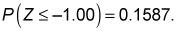Because you're actually looking for the probability that Z is greater than or equal to –1, one more step is required.

Due to the symmetry of the standard normal distribution, the probability that Z is greater than or equal to a negative value equals one minus the probability that Z is less than or equal to the same negative value.

For example,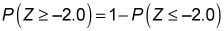This is because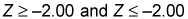are complementary events. This means that Z must either be greater than or equal to –2 or less than or equal to –2. Therefore,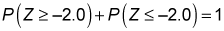This is true because the occurrence of one of these events is certain, and the probability of a certain event is 1.

After algebraically rewriting this equation, you end up with the following result:For the portfolio example,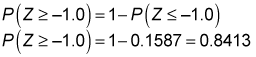The result shows that there's an 84.13 percent chance that the investor's portfolio will have a mean return greater than 8 percent.